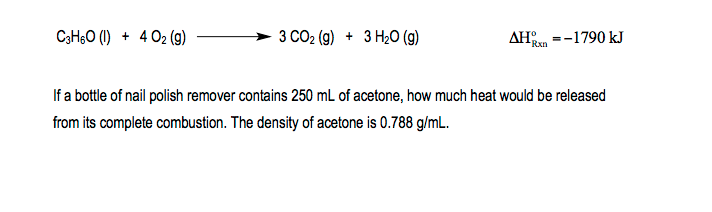# Problem: Consider the following thermochemical reaction for the combustion of acetone, C3H6O, the main component of nail polish remover:

###### FREE Expert Solution
97% (117 ratings)
###### Problem Details

Consider the following thermochemical reaction for the combustion of acetone, C3H6O, the main component of nail polish remover:What scientific concept do you need to know in order to solve this problem?

Our tutors have indicated that to solve this problem you will need to apply the Thermochemical Equation concept. You can view video lessons to learn Thermochemical Equation. Or if you need more Thermochemical Equation practice, you can also practice Thermochemical Equation practice problems.

What is the difficulty of this problem?

Our tutors rated the difficulty ofConsider the following thermochemical reaction for the combu...as medium difficulty.

How long does this problem take to solve?

Our expert Chemistry tutor, Jules took 3 minutes and 15 seconds to solve this problem. You can follow their steps in the video explanation above.

What professor is this problem relevant for?

Based on our data, we think this problem is relevant for Professor Ingram's class at TCU.